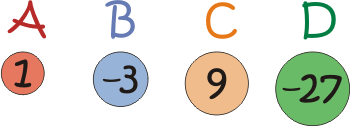#### You may also like### Consecutive Numbers

An investigation involving adding and subtracting sets of consecutive numbers. Lots to find out, lots to explore.### Pair Sums

Five numbers added together in pairs produce: 0, 2, 4, 4, 6, 8, 9, 11, 13, 15 What are the five numbers?### Negative Dice

If the odd numbers on two dice are made negative, which of the totals cannot be achieved?

# Weights

##### Age 11 to 14Challenge LevelImagine you have two of each of the 'weights' above.
Different combinations of the weights available allow you to make different totals.

For example:

$B + C = 6$
$B + 2C = 15$
$A + 2B + C = 4$
$2A + B + 2C + D = -10$

The largest total you can make is $20$ (check you agree).
The smallest total you can make is $-60$ (again, check you agree).

Can you make all the numbers in between?

Is there always a unique way of producing a total, or can different combinations produce the same total?

Extension:

If you are allowed just three different weights this time ($E$, $F$ and $G$), and at least one must be a negative weight, and you are now allowed to have up to three of each, you could choose:

$E = 1$
$F = -4$
$G = 5$

You could make $7$ and $-10$:

$E + F + 2G = 7$
$2E + 3F =$ $-10$

Choose your three weights and test out which totals you can make.

Which set of three weights ($E$, $F$ and $G$) allows you to make the largest range of totals with no gaps in between?

With thanks to Don Steward, whose ideas formed the basis of this problem.